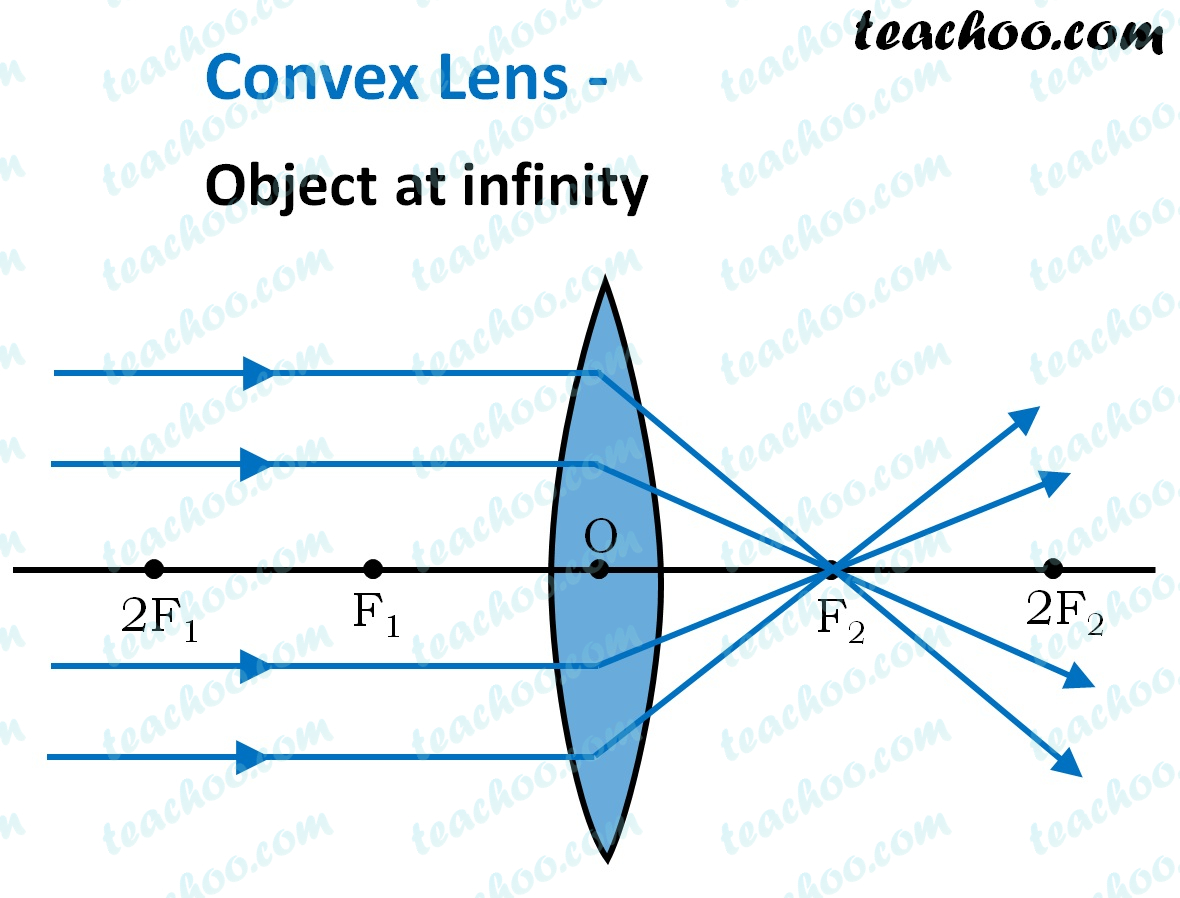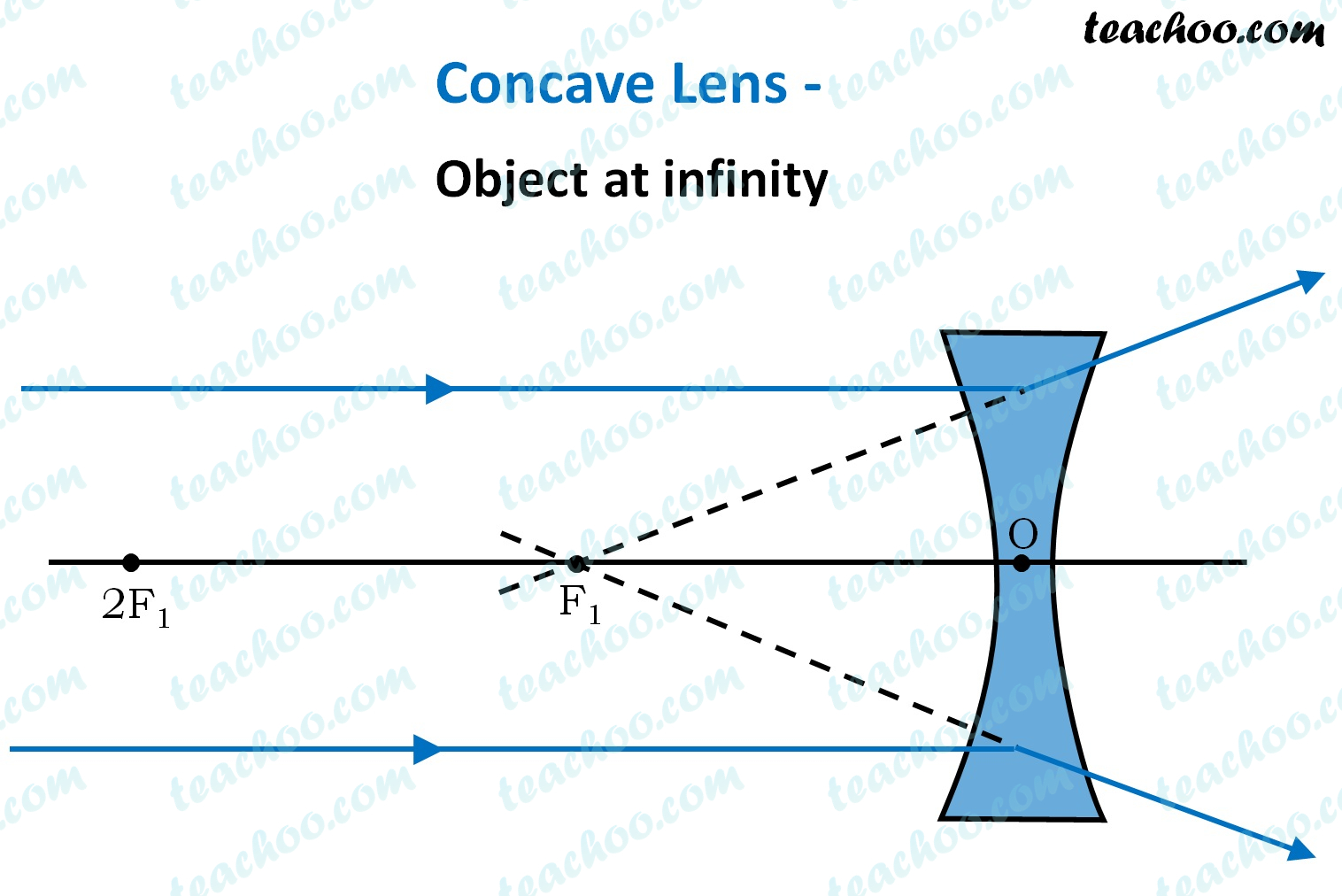Concepts

Class 10
Chapter 10 Class 10 - Light - Reflection and Refraction

## Optical Center (O)

It is the Center Point of Lens

It is denoted by letter O

## Principal Axis

It is the line passing through Center of Lens

It is perpendicular to both faces of lens

## Principal Focus (F) of Convex Lens

The point at which

rays parallel to principal axis

coming from infinity converge

after being refracted from Convex Lens

is called the principal focus of Convex Lens## Principal Focus (F) of Concave Lens

The point at which

rays parallel to principal axis

coming from infinity appear to converge

after being refracted from Concave Lens

is called the principal focus of Concave LensNote : - Here we assume ray of light falls from left to right

It is also possible that ray of light falls from right to left

In this case, these lines will converge on other side of lens

This point will be called F'

Hence there are 2 focus (F and F')

(More than 1 focus is called foci)

## Focal Length

It is distance between Optical Center (O) and Focus (F)

It is represented by the letter f (small letter f)

Learn in your speed, with individual attention - Teachoo Maths 1-on-1 Class